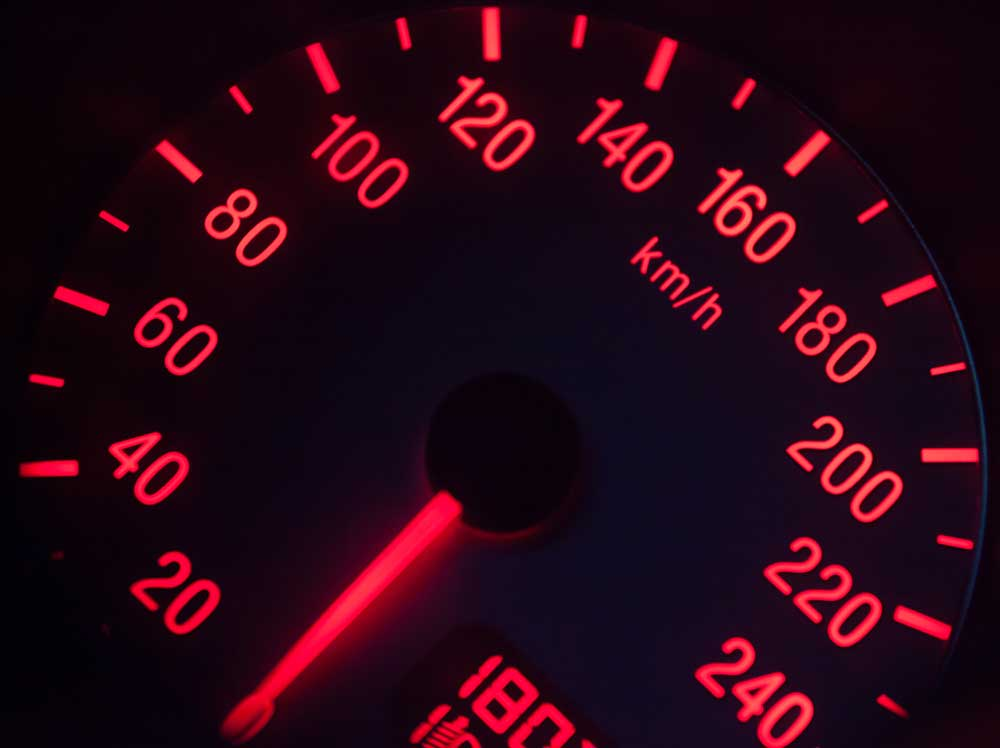# Speed Conversion CalculatorsConvert one unit of speed to another. Start by selecting the speed unit to convert from.

## What is SpeedSpeed is a measurement of how fast an object is moving, or the rate that an object moves over a distance. An object moving at a high speed will cover a lot of distance in a short amount of time, while an object moving at a low speed will cover little distance over a longer amount of time.

Thus, speed can be measured as the distance an object travels divided by the time it takes to travel this distance, ie. speed = distance / time.

Speed over land is commonly measured in miles per hour or kilometers per hour, and speed over sea is commonly measured in knots. The speed of sound is equal to 767.27 miles per hour or 1,235 kilometers per hour.

## Units of Speed Measurement

Units of speed include knots, Mach number, speed of sound, speed of light, miles per hour, feet per second, kilometers per second, kilometers per hour, meters per second, and centimeters per second.

## Common Speed Measurement Conversions

Knots to miles per hour speed conversion
1 knot is equal to 1.150779 miles per hour
Miles Per Hour to kilometers per hour speed conversion
1 mile per hour is equal to 1.609344 kilometers per hour
Mach Number to miles per hour speed conversion
1 Mach is equal to 767.269148 miles per hour
Mach Number to kilometers per hour speed conversion
1 Mach is equal to 1,235 kilometers per hour
Speed Of Sound to miles per hour speed conversion
1 speed of sound is equal to 767.269148 miles per hour
Meters Per Second to feet per second speed conversion
1 meter per second is equal to 3.28084 feet per second

## Speed Unit Conversion Table

Equivalent speed metric and imperial measurements
Convert From Knot Mach Speed Of Sound Speed Of Light Mile Per Hour Foot Per Second Kilometer Per Second Kilometer Per Hour Meter Per Second Centimeter Per Second
1 knot = 1 kn Mach 0.0015 0.0015 sound 0.000000001716 c 1.150779 mph 1.68781 ft/s 0.000514 km/s 1.852 km/h 0.514444 m/s 51.444444 cm/s
Mach 1 = 666.738661 kn Mach 1 1 sound 0.0000011441 c 767.269148 mph 1,125 ft/s 0.343 km/s 1,235 km/h 343 m/s 34,300 cm/s
speed of sound = 666.738661 kn Mach 1 1 sound 0.0000011441 c 767.269148 mph 1,125 ft/s 0.343 km/s 1,235 km/h 343 m/s 34,300 cm/s
speed of light = 582,749,918 kn Mach 874,030 874,030 sound 1 c 670,616,629 mph 983,571,056 ft/s 299,792 km/s 1,079,252,849 km/h 299,792,458 m/s 29,979,245,800 cm/s
1 mile per hour = 0.868976 kn Mach 0.001303 0.001303 sound 0.0000000014912 c 1 mph 1.466667 ft/s 0.000447 km/s 1.609344 km/h 0.44704 m/s 44.704 cm/s
1 foot per second = 0.592484 kn Mach 0.000889 0.000889 sound 0.0000000010167 c 0.681818 mph 1 ft/s 0.000305 km/s 1.09728 km/h 0.3048 m/s 30.48 cm/s
1 kilometer per second = 1,944 kn Mach 2.915452 2.915452 sound 0.0000033356 c 2,237 mph 3,281 ft/s 1 km/s 3,600 km/h 1,000 m/s 100,000 cm/s
1 kilometer per hour = 0.539957 kn Mach 0.00081 0.00081 sound 0.00000000092657 c 0.621371 mph 0.911344 ft/s 0.000278 km/s 1 km/h 0.277778 m/s 27.777778 cm/s
1 meter per second = 1.943844 kn Mach 0.002915 0.002915 sound 0.0000000033356 c 2.236936 mph 3.28084 ft/s 0.001 km/s 3.6 km/h 1 m/s 100 cm/s
1 centimeter per second = 0.019438 kn Mach 0.000029155 0.000029155 sound 0.000000000033356 c 0.022369 mph 0.032808 ft/s 0.00001 km/s 0.036 km/h 0.01 m/s 1 cm/s